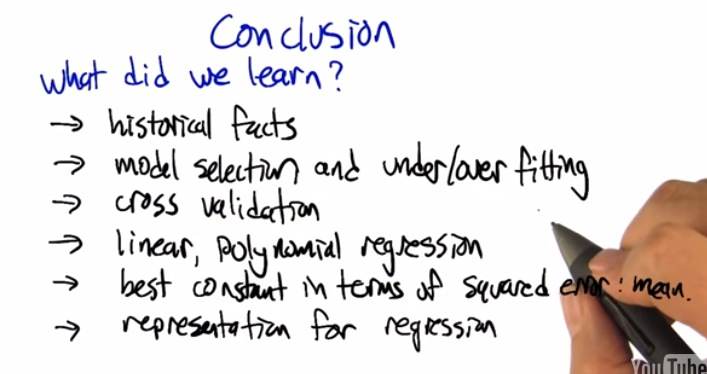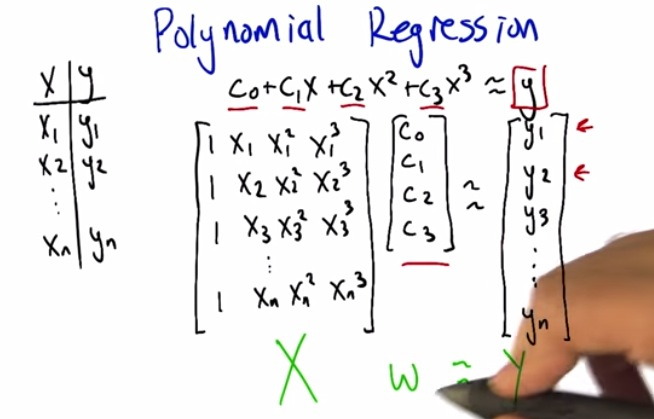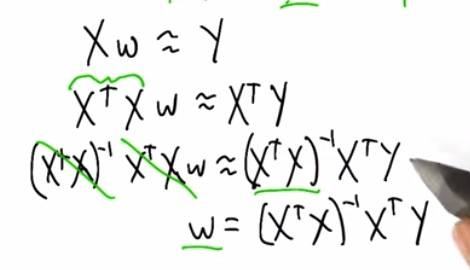• Inverse can't be implemented directly as X is not a squared matrix. That's way XtX to produce square matrix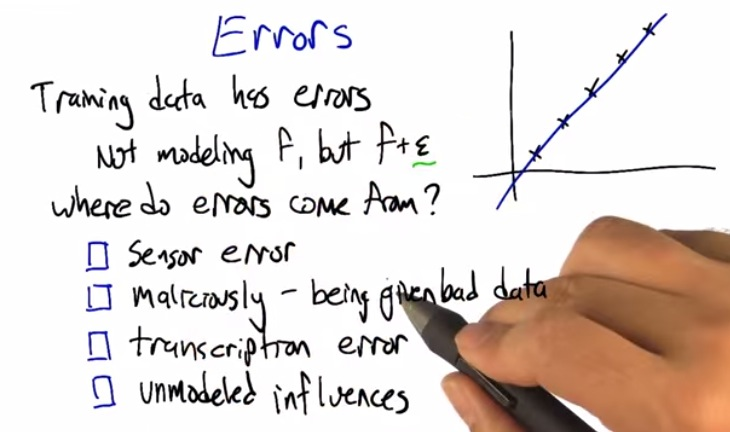• So sensor could be comes from many kinds
• Sensor error: The machine incorreclty sense the input and thus giving a bad input
• Maliciously, data is wrong interpreted. As we want to gather big data comes from many different resources, collecting it into one database could often lead to bad data.
• Transcription error: in the case of human error, collecting bad data
• Unmodeled influences: we might ourself wrong to model our problem. As shown in predicting the house cost, size along couldn't measure the price of a house. Could be (location,n number bedroom, etc.)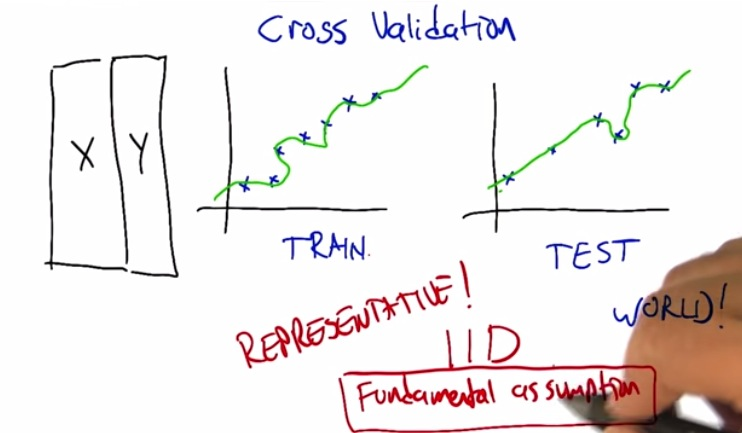• The data that drawn from train,cv,and test set should be IID (independently identical data). And thus assume all dataset come from same normal distribution.
• That  is the fundamental assumption
• We want to test our function that gather from train set to test set. Train from test set could not gain anything useful, as we want our function to generalized upcoming data.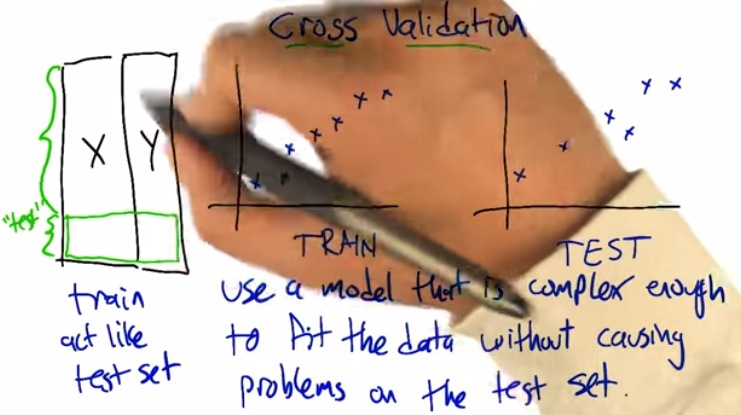• After we build our model from train set, we want to try order of polynomial to see if it has better fit.
• In order to use it we need more dataset. Using it on test set could once again consider cheating
• What we could do is splitting our train set to give to cross validation set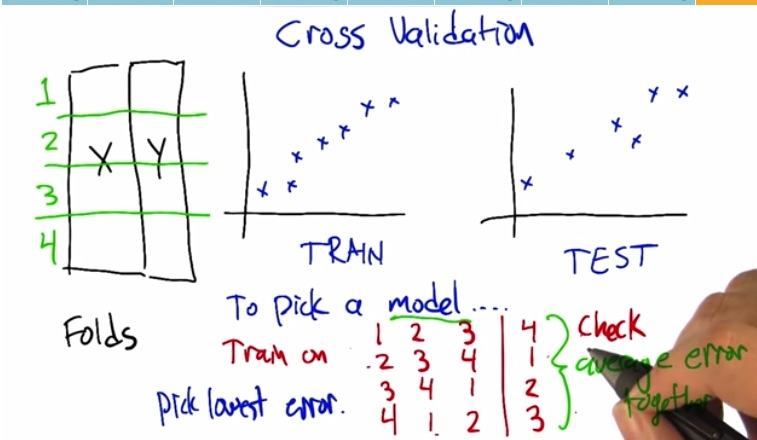• Then for split our data, and try many order of polynomial .
• From the table each attribute assigned with each order of polynomial .
• The result then picked model with the lowest cross validation error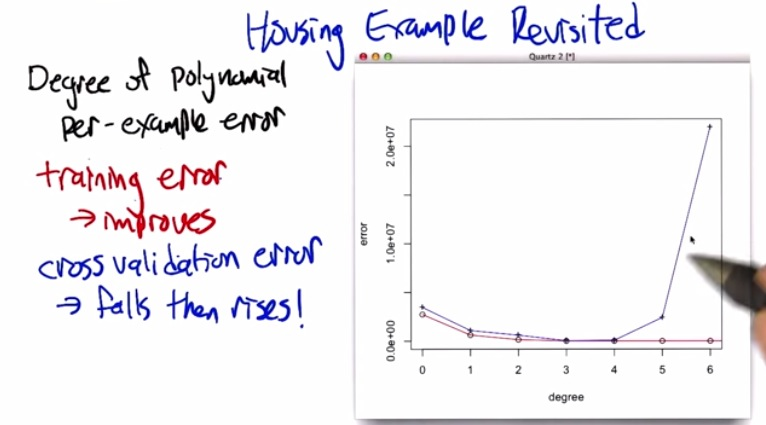• Each chunks of the data is test on the cross validation set.
• Each chunks assigned by different order of polynomial , and test on the cv.
• Average the error of each chunks, and pick order of polynomial with lowest cross validation error.
• Now, the graph shown on the red, is the error rate. As we increase the order of polynomial , its error down with degree 3 the threshold, then same error train
• Then the blue line denote by we testing the our function with our order of polynomial . Here we see as it decreases, the cv rise again as order of polynomial increases.
• As we learned in machine learning, increasing the order of polynomial will be overmatch the existing data, try it to the cv, give us high error.Order of 3 and 4 give some generalization that can handle train set and cv set very well.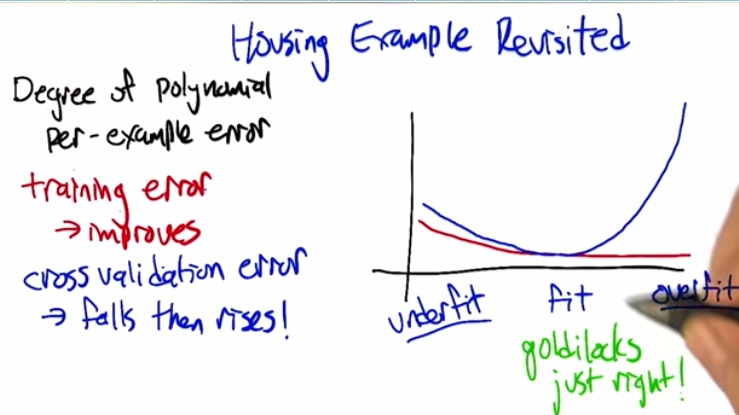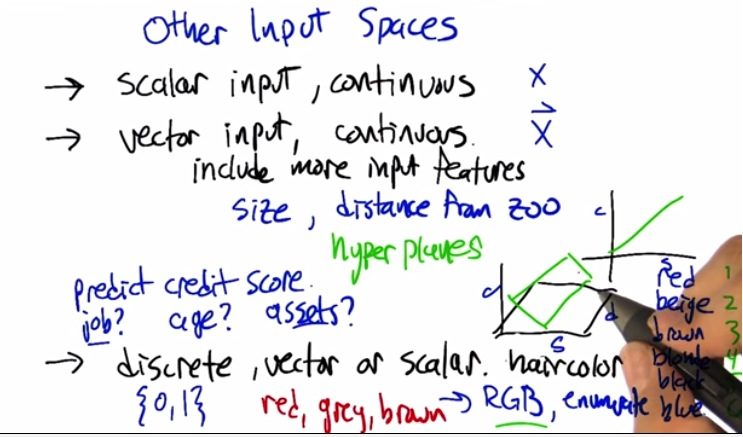• Input can be scalar/vector
• To predict credit score, given job,age,asset,other continuous input. We can take it as boolean value. In hair cases, enumerate, take rgb number (higher rgb, higher the score)